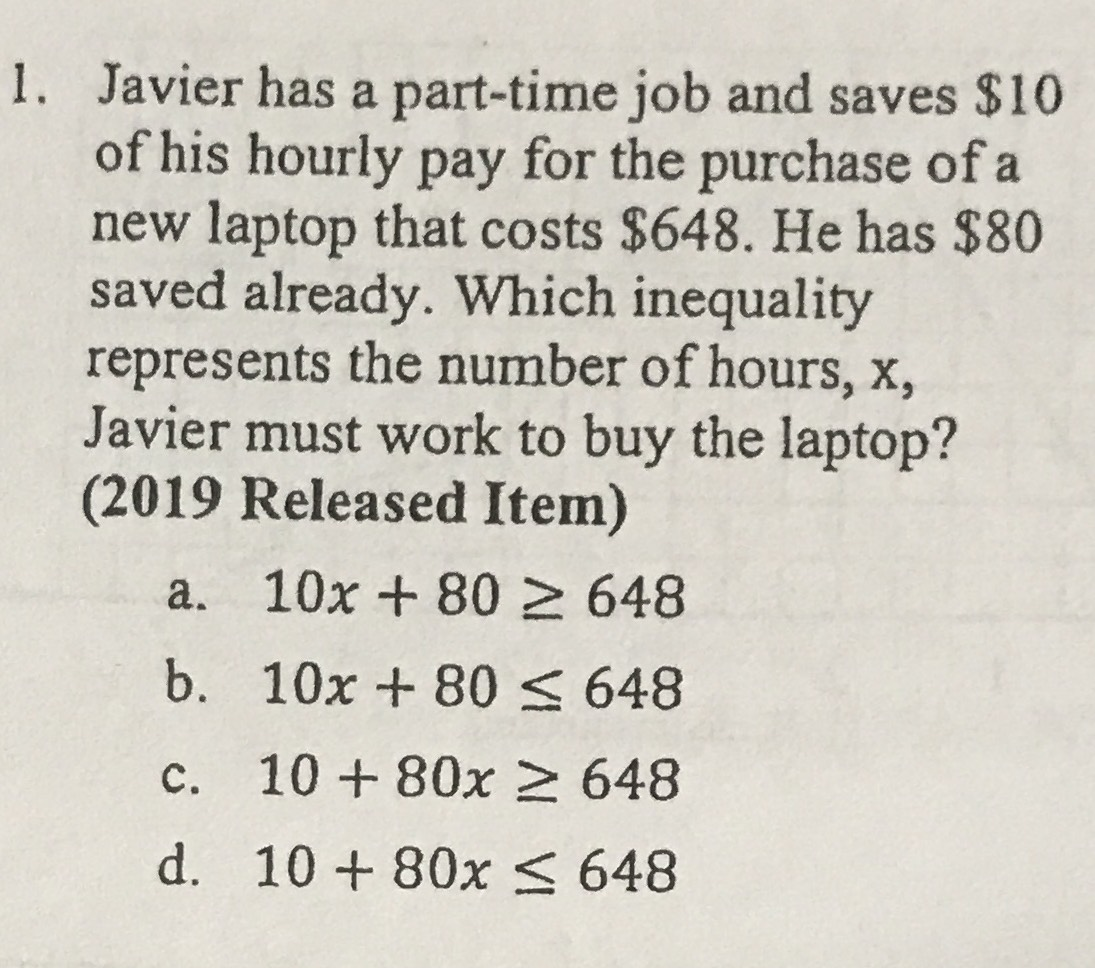### ¿Todavía tienes preguntas de matemáticas?

Pregunte a nuestros tutores expertos
Algebra
Pregunta1. Javier has a part-time job and saves $$\ 10$$ of his hourly pay for the purchase of a new laptop that costs $$\ 648$$ . He has $$\ 80$$ saved already. Which inequality represents the number of hours, $$x ,$$ Javier must work to buy the laptop? (2019 Released Item)

a. $$10 x + 80 \geq 648$$

b. $$10 x + 80 \leq 648$$

c. $$10 + 80 x \geq 648$$

d. $$10 + 80 x \leq 648$$